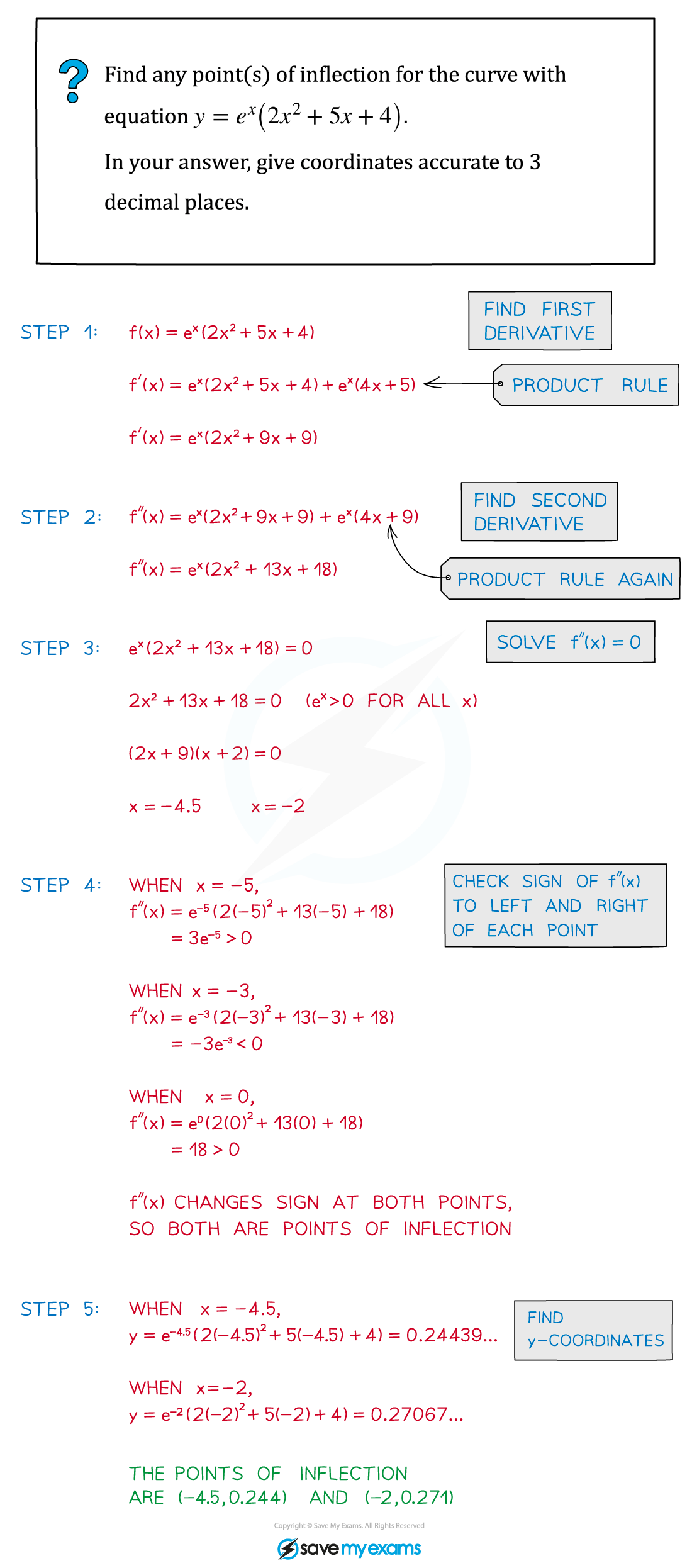# AQA A Level Maths: Pure复习笔记7.4.2 Points of Inflection

### Points of Inflection

#### What is a point of inflection?

• At AS level you encountered points of inflection when discussing stationary points
• When the sign of the first derivative (ie of the gradient) is the same on both sides of a stationary point, then the stationary point is a point of inflection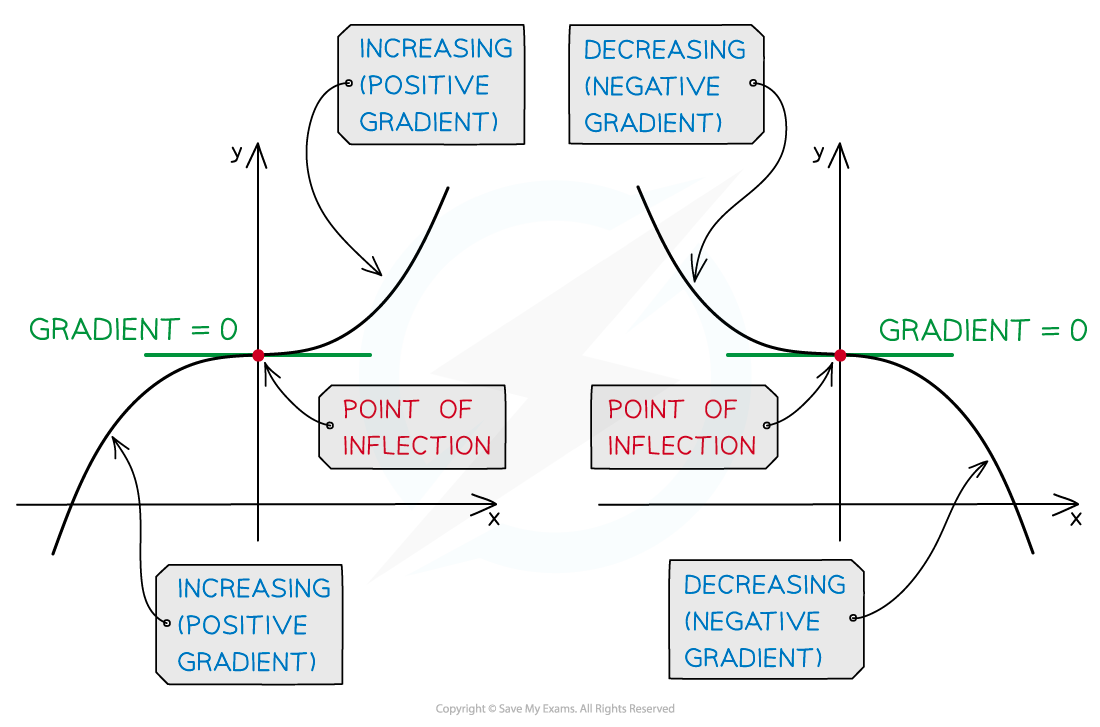• A point of inflection does not have to be a stationary point however
• A point of inflection is any point at which a curve changes from being convex to being concave
• This means that a point of inflection is a point where the second derivative changes sign (from positive to negative or vice versa)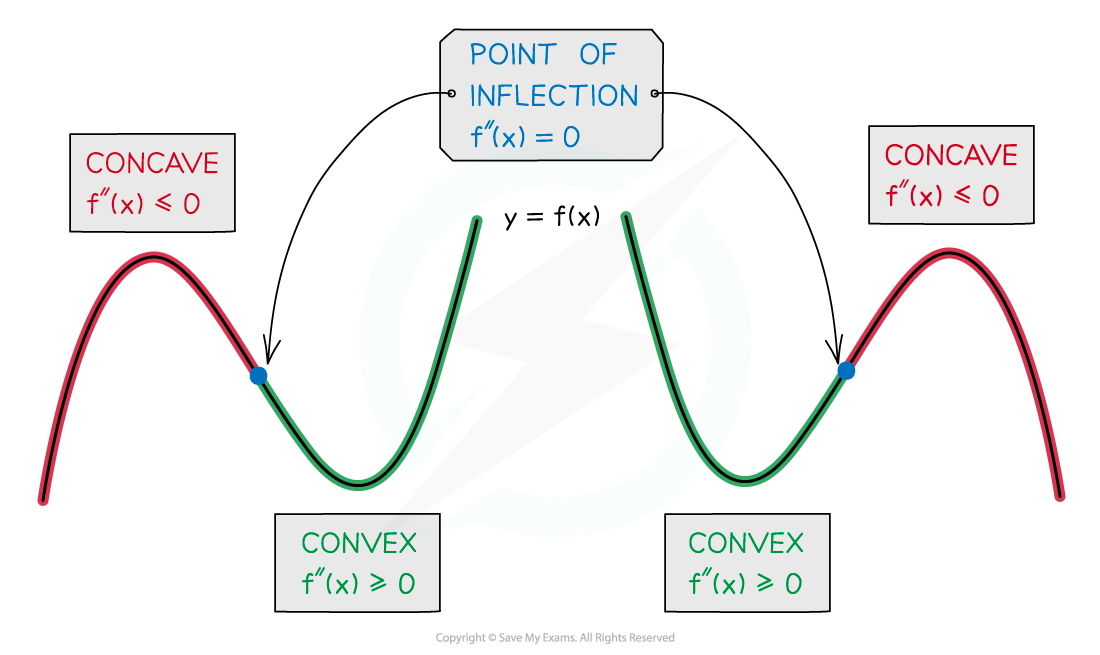• To find the points of inflection of a curve with equation y = f(x):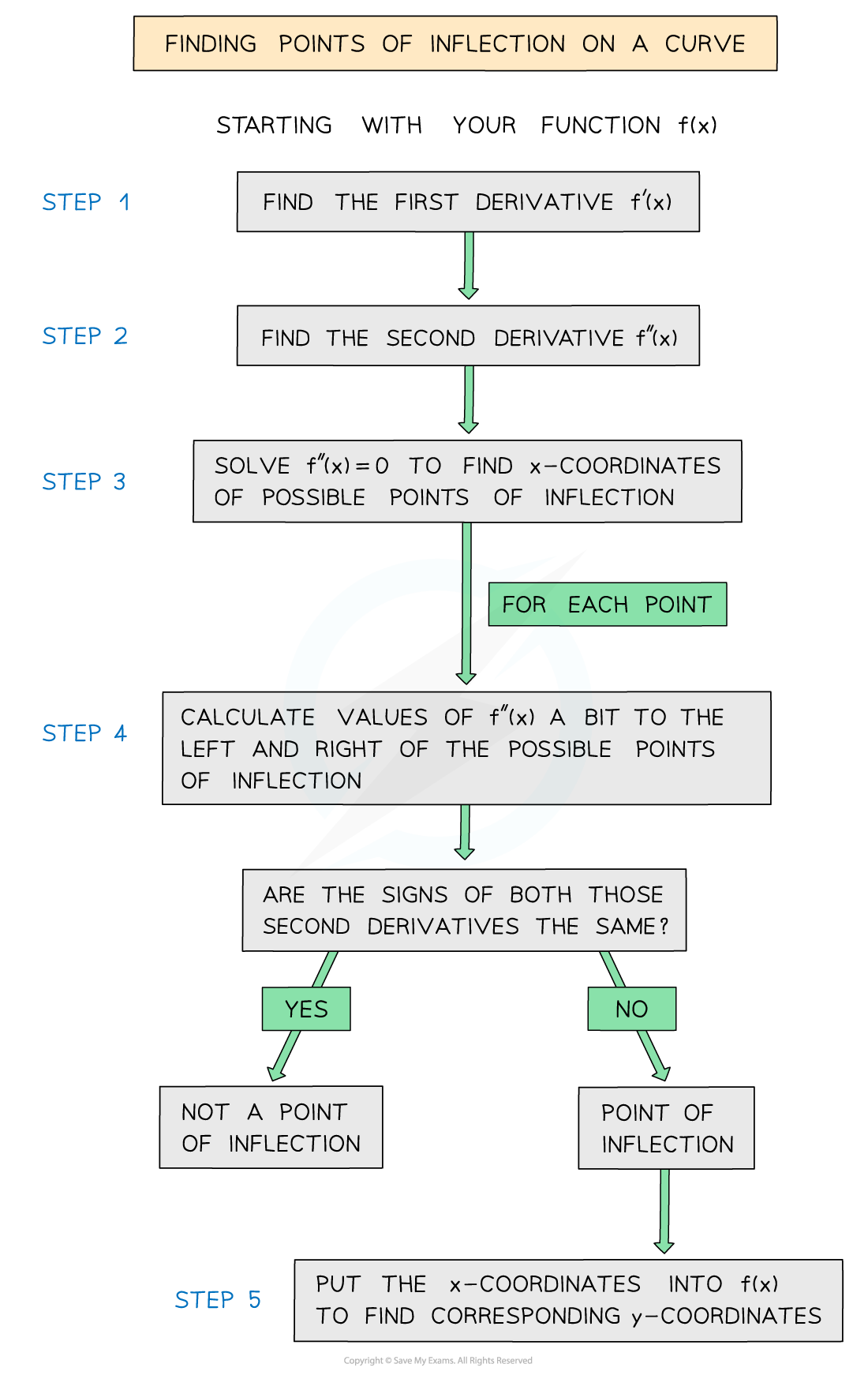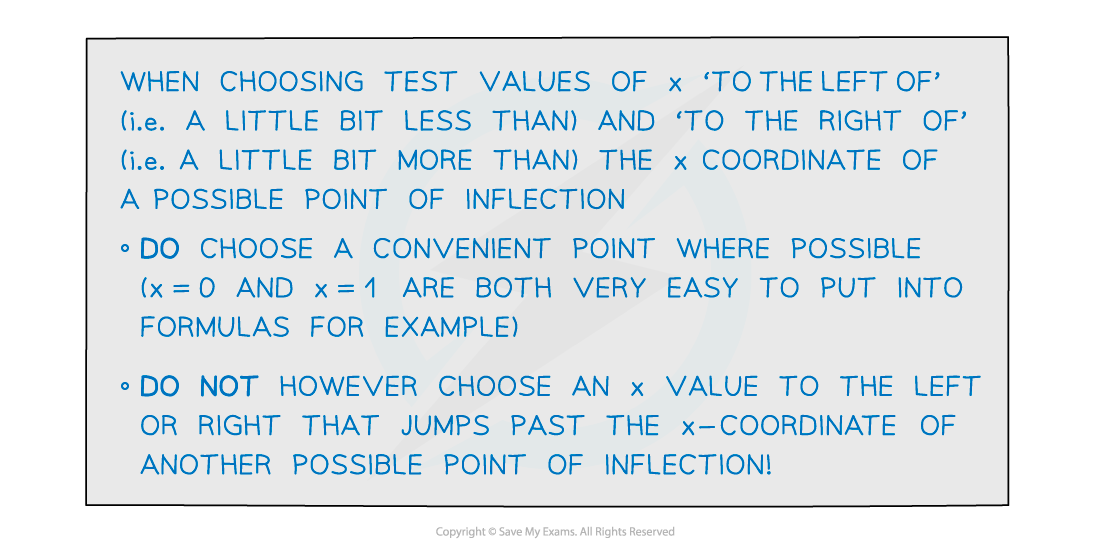#### Exam Tip

• Remember – the first derivative (ie the gradient) does NOT have to be zero at a point of inflection!

#### Worked Example